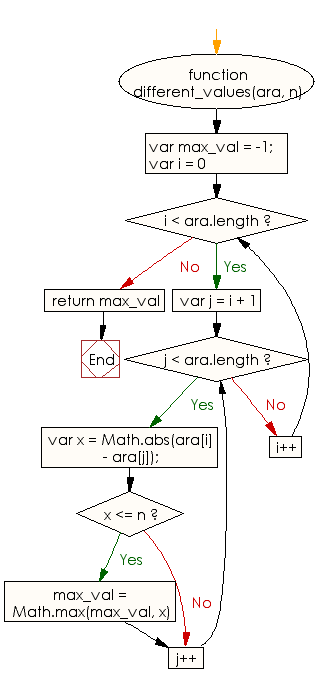# JavaScript: Find two elements of the array such that their absolute difference is not greater than a given integer but is as close to the said integer

## JavaScript Basic: Exercise-104 with Solution

Write a JavaScript program to find two elements of the array such that their absolute difference is not greater than a given integer but is as close to the said integer.

Sample Solution:

HTML Code:

``````<!DOCTYPE html>
<html>
<meta charset="utf-8">
<meta name="viewport" content="width=device-width">
<title> Find two elements of the array such that their absolute difference is not greater than a given integer but is as close to the said integer</title>
<body>

</body>
</html>
```
```

JavaScript Code:

``````function different_values(ara, n) {
var max_val = -1;
for (var i = 0; i < ara.length; i++) {
for (var j = i + 1; j < ara.length; j++) {
var x = Math.abs(ara[i] - ara[j]);
if (x <= n) {
max_val = Math.max(max_val, x)
}
}
}
return max_val
}
console.log(different_values([12, 10, 33, 34], 10));
console.log(different_values([12, 10, 33, 34], 24));
console.log(different_values([12, 10, 33, 44], 40));
``````

Sample Output:

```2
24
34
```

Flowchart:ES6 Version:

``````function different_values(ara, n) {
let max_val = -1;
for (let i = 0; i < ara.length; i++) {
for (let j = i + 1; j < ara.length; j++) {
const x = Math.abs(ara[i] - ara[j]);
if (x <= n) {
max_val = Math.max(max_val, x)
}
}
}
return max_val
}
console.log(different_values([12, 10, 33, 34], 10));
console.log(different_values([12, 10, 33, 34], 24));
console.log(different_values([12, 10, 33, 44], 40));
``````

Live Demo:

See the Pen javascript-basic-exercise-104 by w3resource (@w3resource) on CodePen.

What is the difficulty level of this exercise?

Test your Programming skills with w3resource's quiz.

﻿

## JavaScript: Tips of the Day

Checks if a string is an anagram of another string (case-insensitive, ignores spaces, punctuation and special characters)

Example:

```const isAnagram = (str1, str2) => {
const normalize = str =>
str
.toLowerCase()
.replace(/[^a-z0-9]/gi, '')
.split('')
.sort()
.join('');
return normalize(str1) === normalize(str2);
};
console.log(isAnagram('iceman', 'cinema')); // true
```

Output:

```true
```